# GMAT Math : Understanding absolute value

## Example Questions

1 2 4 Next →

### Example Question #31 : Understanding Absolute Value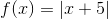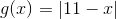If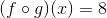, then how many possible values ofare there?

Zero

Four

Two

Three

One

Two

Explanation:can be rewritten as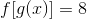, so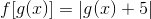.

If, then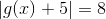, or, equivalently, either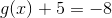or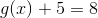.

Solve separately: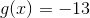or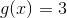, so the above two statements can be rewritten as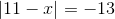and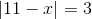has no solution, since the absolute value of a number cannot be negative.can be rewritten as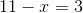and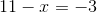It is not necessary to solve these statements, as we can determine that the correct response is two solutions.

### Example Question #32 : Absolute Value

Solve for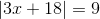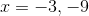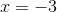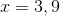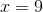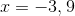Explanation:

To solve absolute value equations, we must set up two equations: one where the solution is negative, and one where the solution is positive.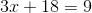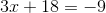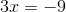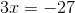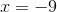### Example Question #33 : Absolute Value

True or false:is a positive number.

Statement 1: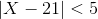Statement 2: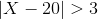STATEMENT 1 ALONE provides sufficient information to answer the question, but STATEMENT 2 ALONE does NOT provide sufficient information to answer the question.

BOTH STATEMENTS TOGETHER provide sufficient information to answer the question, but NEITHER STATEMENT ALONE provides sufficient information to answer the question.

EITHER STATEMENT ALONE provides sufficient information to answer the question.

BOTH STATEMENTS TOGETHER do NOT provide sufficient information to answer the question.

STATEMENT 2 ALONE provides sufficient information to answer the question, but STATEMENT 1 ALONE does NOT provide sufficient information to answer the question.

STATEMENT 1 ALONE provides sufficient information to answer the question, but STATEMENT 2 ALONE does NOT provide sufficient information to answer the question.

Explanation:

Assume Statement 1 alone.can be rewritten as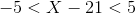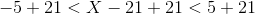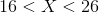Therefore,is positive.

Assume Statement 2 alone. The sign ofcannot be determined. For example, if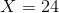, which is positive, then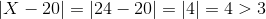.

If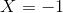, which is not positive, then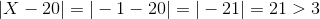.

### Example Question #34 : Absolute Value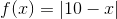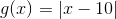How many values ofmake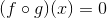a true statement?

Four

Two

Three

One

None

Two

Explanation: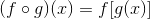, so we want the number of values offor which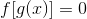., so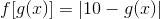Therefore, if, then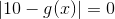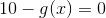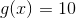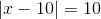Either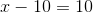, in which case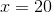, or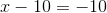, in which case.

The correct choice is therefore two.

### Example Question #35 : Absolute Value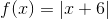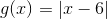How many values ofmake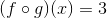a true statement?

Two

None

One

Three

Four

None

Explanation:, so we want the number of values offor which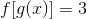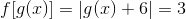, so either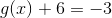or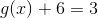If the first equation is true, then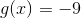and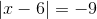.

If the second equation is true, then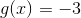and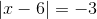.

In each situation, the absolute value of an expression would be negative; since the absolute value of an expression cannot be negative, no solution is yielded.

There are no values ofthat maketrue; the correct response is zero.

1 2 4 Next →

Tired of practice problems?

Try live online GMAT prep today.